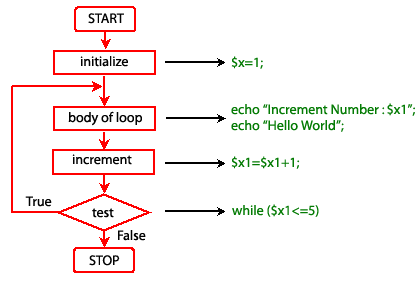# PHP do while Loop Statement

## Description

There is a small difference between the while and do while loops. The difference is the place where the condition is tested. As we already seen in a while loop that condition is tested at the beginning, i.e. before execution any of the statement within the while loop.

In the case of do while loop the condition is tested after having executed the statements within the loop. This means that do-while would execute its statements at least once, even if the condition fails for the first time itself.

Syntax:

```do
{
execute the statements;
}
while (condition is true)
```

Example -1:

The following example print "Increment Number" with \$x1 value as well as "Hello world" five times.

``````<?php
\$x1=1;
do {
echo "Increment Number : \$x1 <br />";
echo "Hello World  <br />";
\$x1=\$x1+1;
}while (\$x1<=5)
?>``````

Output:

```Increment Number : 1
Hello World
Increment Number : 2
Hello World
Increment Number : 3
Hello World
Increment Number : 4
Hello World
Increment Number : 5
Hello World```

View the example in the browser

Pictorial representation of do while loopExample -2:

In the following example, the loop will run one time exactly since after the first iteration, when truth expression is checked, it evaluates to FALSE (as \$X is not bigger than 5) and the loop execution ends.

``````<?php
\$x1=0;
do {
echo "Increment Number : \$x1 <br />";
echo "Hello World <br />";
\$x1=\$x1+1;
}while (\$x1>5)
?>``````

Output:

```Increment Number : 0
Hello World ```

Previous: while statement
Next: for statement

﻿

## PHP: Tips of the Day

Members of objects or classes can be accessed using the object operator (->) and the class operator (::).

Example:

```class MyClass {
public \$a = 1;
public static \$b = 2;
const C = 3;
public function d() { return 4; }
public static function e() { return 5; }
}
\$object = new MyClass();
var_dump(\$object->a); // int(1)
var_dump(\$object::\$b); // int(2)
var_dump(\$object::C); // int(3)
var_dump(MyClass::\$b); // int(2)
var_dump(MyClass::C); // int(3)
var_dump(\$object->d()); // int(4)
var_dump(\$object::d()); // int(4)
var_dump(MyClass::e()); // int(5)
\$classname = "MyClass";
var_dump(\$classname::e()); // also works! int(5)
```

Note that after the object operator, the \$ should not be written (\$object->a instead of \$object->\$a). For the class operator, this is not the case and the \$ is necessary. For a constant defined in the class, the \$ is never used.

Also note that var_dump(MyClass::d()); is only allowed if the function d() does not reference the object:

```class MyClass {
private \$a = 1;
public function d() {
return \$this->a;
}
}
\$object = new MyClass();
var_dump(MyClass::d()); // Error!
```

This causes a 'PHP Fatal error: Uncaught Error: Using \$this when not in object context'

These operators have left associativity, which can be used for 'chaining':

```class MyClass {
private \$a = 1;

\$this->a += \$a;
return \$this;
}

public function get() {
return \$this->a;
}
}
\$object = new MyClass();
```

These operators have the highest precedence (they are not even mentioned in the manual), even higher that clone. Thus:

```class MyClass {
private \$a = 0;
\$this->a += \$a;
return \$this;
}
public function get() {
return \$this->a;
}
}
\$o1 = new MyClass();
var_dump(\$o1->get()); // int(2)
var_dump(\$o2->get()); // int(2)
```

The value of \$o1 is added to before the object is cloned!

Note that using parentheses to influence precedence did not work in PHP version 5 and older (it does in PHP 7):

```// using the class MyClass from the previous code
\$o1 = new MyClass();
\$o2 = (clone \$o1)->add(2); // Error in PHP 5 and before, fine in PHP 7
var_dump(\$o1->get()); // int(0) in PHP 7
var_dump(\$o2->get()); // int(2) in PHP 7
```